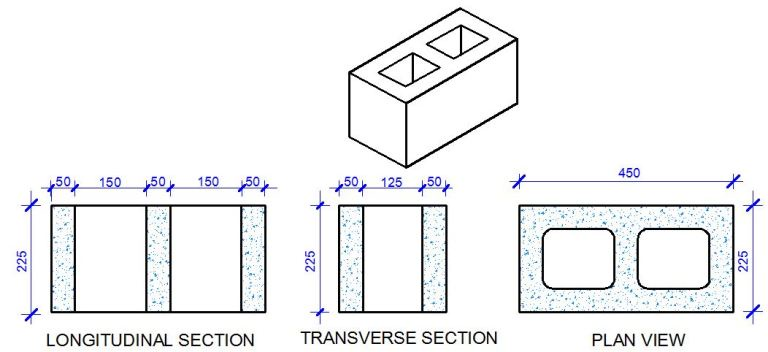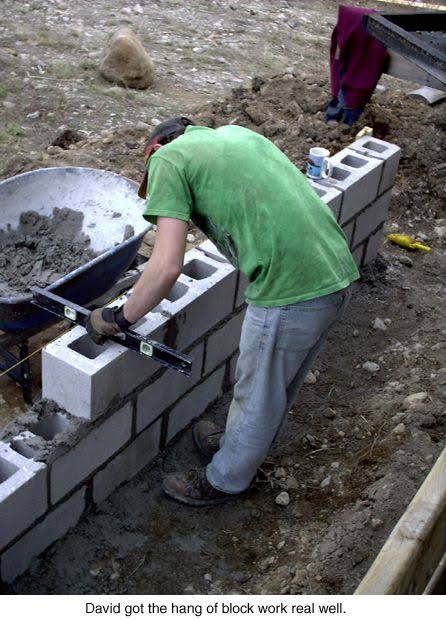Review

# How to Calculate Bags of Cement and Tons of Sand for Block Laying

###How to Calculate Bags of Cement and Tons of Sand for Block LayingIn construction industry today, Engineer or the Artisans give their clients wrong quotation of Bags of Cement and Tons of Sand needed for the construction of building because they know that the clients are novice. This is a great opportunity for everyone of us to learn this simple calculation in order not to be scammed by anybody.Always Know that 10 Blocks is required to Build 1m² of Wall.

Proof:

From the Image above, Square meter for a Block = 450mm x 225mm = 0.101 m2

Now, Total Number of Blocks required for 1m² = 1/0.101 = 9.99 (say 10 blocks)

Making use of the Concrete Block Dimension Above with 25mm of Mortar Thickness, We can State the Volume of Mortal For 1m² Wall:Volume of Horizontal Mortar = 10 Blocks × ( 0.45m × 0.225m × 0.025m) = 0.0159 m3

Volume of Vertical Mortar = 8 Lines × ( 0.225m × 0.225m × 0.025m) = 0.0101 m3

Total Volume of Mortar Required for Laying 1m² of Block = 0.0159 + 0.0101 = 0.026m³

Therefore the volume of mortar required to lay one square metre of 9 inches block (with hole) can be taken as 0.026m3 for all practical purposes.

let us assume that we have 100m2 of wall, and we want to estimate the quantity of cement and sand for mortar needed to lay the blocks.

The volume of mortar required = 0.026 x 100 = 2.6 m3

Mix ratio for mortar (laying of blocks) is 1:6

#### CEMENT

Addition of the Mix Ratio = 7

Density of 1 bag of Cement = 1440kg/m³

Quantity of cement required = 1/7 x 1440 kg/m3 = 205.71 kg

Making allowance for Buckling between fresh and wet Mortar = 1.35 x 205.71 = 277.71 kg

Quantity of cement required in bags = 277.71/50 = 5.55 bags

5.55 bags of Cements = 1m³ of Mortar

For 2.6m³ which Cover 100m² of Concrete Blocks

5.55 bags of Cements = 1m³ of Mortar

X bags of Cements = 2.6m³ of Mortar

X = 14.43 bags of Cement

14.43 Bags of Cement is Required for Laying of 100m² Concrete Block.

#### SAND

Addition of the Mix Ratio = 7

Density of Sand = 1600kg/m³

Quantity of Sand required = 6/7 x 1600 kg/m3 = 1371.428 kg

Making allowance for Buckling between fresh and wet Mortar = 1.35 x 1371.428 = 1851.43 kg

1.85 Tons of Sand = 1m³ of Mortar

For 2.6m³ which Cover 100m² of Concrete Blocks

1.85 Tons of Sand = 1m³ of Mortar

X Tons of Sand = 2.6m³ of Mortar

X = 4.6 Tons of Sand

4.6 Tons of Sand is Required for Laying of 100m² Concrete Block.